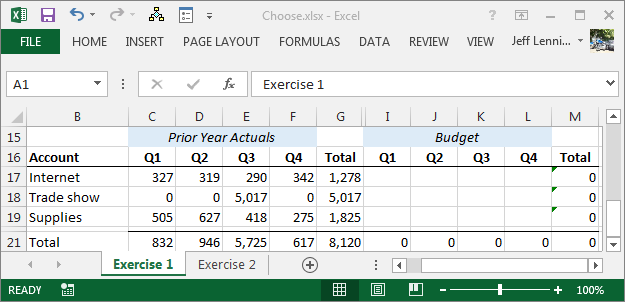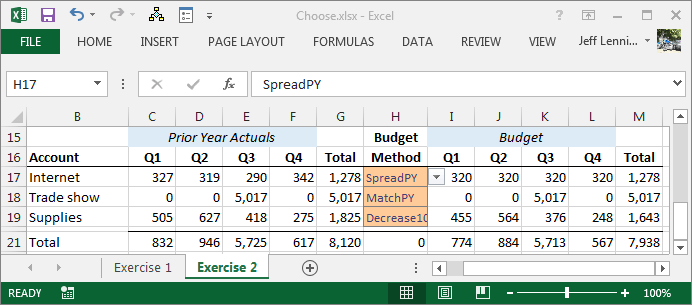## Pick the Math with Excel’s CHOOSE Function

Have you ever wanted to allow the workbook user to pick the math that a formula should use?  This post explores the CHOOSE function, which, among other things, enables us to allow the user to pick the math.

## CHOOSE

Before jumping right into the function’s syntax, let’s get a little context.  Let’s say that we have a budget worksheet.  It contains prior year actual values, and we want the user (our department manager) to prepare the budget for next year.  This can be visualized with the screenshot below.We want to make this process as efficient as possible, and thus, we want to make it fast and easy for our user.  Rather than require our manager to type in budget amounts for each account, we decide to allow the budget manager to select a budget method for each account and let Excel perform the relevant math.  For example, we want to supply a budget method that will take prior actual amounts and spread them out evenly for the budget year.  This method is perfect for small accounts.  We also want a method that will match prior year amounts exactly, because some of the accounts are cyclical in nature, for example, the annual trade show.  Lastly, we want to provide a method that cuts prior year amounts by 10%.

We want the budget manager to walk through each account and assign the most appropriate budget method.  Based on the selected budget method, we want Excel to compute the math.

This type of set up is made easy with the CHOOSE function. The function’s syntax follows:

=CHOOSE(index_num, value1, [value2], …)

Where:

• index_num is expressed as an integer, and tells the function which argument to evaluate and return.  If index_num equals 1, then the value1 argument is returned.  If index_num equals 2, then the value2 argument is returned, and so on.
• value1 is the argument to return if index_num equals 1.  Please note this argument can be expressed as a value, cell reference, function, or formula.
• [value2] is optional, and will be returned if index_num equals 2
• … and so on, up to about 254 arguments

## Budget Methods

The idea then is to allow the user to control the index_num argument, and depending on its value the CHOOSE function will compute the proper amount.  For example, let’s say that the user can enter the budget method into cell A1. Let’s assume the spread prior year (SpreadPY) budget method is code 1, that the match prior year (MatchPY) method is code 2, and that the decrease prior year by 10% (Decrease10) method is code 3.  Conceptually, we could set up a formula to compute the proper budget amount based on the budget method:

Where:

• A1 is the cell that stores the user-entered budget method
• SpreadPY represents the formula that computes the proper amount
• MatchPY represents the formula that computes the proper amount
• Decrease10 represents the formula that computes the proper amount

This idea is illustrated in the screenshot below, where the user enters the budget method in column H, and the budget cells are computed by the CHOOSE function.The formula for I17, which is filled throughout the budget range (I17:L:19), follows:

=CHOOSE(\$H17,\$G17/4,C17,C17*0.9)

Where:

• \$H17 is the cell that contains the desired budget method
• \$G17/4 is the formula for code 1, the SpreadPY method.  This takes the sum of last year and divides it by 4.
• C17 is the formula for code 2, the MatchPY method.  This takes the value in the prior year cell to match each quarter’s value.
• C17*0.9 is the formula for code 3, the Decrease10 method.  This takes the value in the prior year cell and multiplies it by 90%, effectively giving it a 10% decrease.

The variety of budget methods that could be set up are only limited by our imagination.  We could set up a zero method that sets each cell to zero.  We could set up a method that prepares the budget based on headcount, basically taking last year actual values divided by last year headcount, and multiplying the result by the budget year’s headcount.  We could also set up a method for manual.  Where the user enters the manual budget items into a separate worksheet, and the method simply retrieves the values from this manual input area via a lookup or conditional summing function.  Since the budget method formula is simply any Excel formula, the possibilities are endless.

## Next Level

You could certainly build on this idea, and take it to the next level. For example, if you are comfortable with Data Validation and the Match function, you could allow the user to pick the budget method from an in-cell dropdown, alleviating the need for the user to memorize the budget method codes, as illustrated in the attached file.If you are comfortable with named formulas, you could create a name for each budget method formula, so that the CHOOSE function’s arguments are names instead of formulas, simplifying the workbook and making it more elegant and modular.

Well, those are some ideas for how to incorporate the CHOOSE function into your workbooks.  Here, we let the user choose the math for a budget workbook, but this idea can be used beyond the budget illustration presented.

If you’d like, feel free to check out the Choose workbook used to create the screenshots above since it contains the formulas.

Excel rules!

Posted in ,### Jeff Lenning

I love sharing the things I've learned about Excel, and I built Excel University to help me do that. My motto is: Learn Excel. Work Faster.